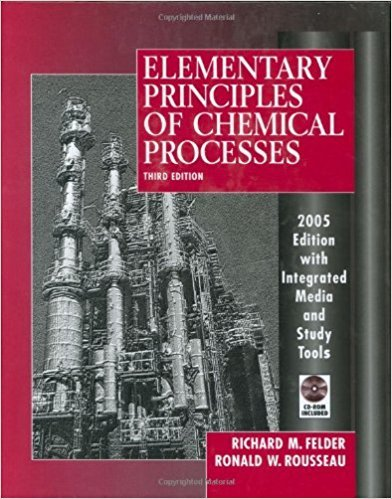×
Get Full Access to Elementary Principles Of Chemical Processes - 3 Edition - Chapter 14 - Problem 14.18
Get Full Access to Elementary Principles Of Chemical Processes - 3 Edition - Chapter 14 - Problem 14.18

×

# We assumed a 39% efficiency for this power plant, meaningISBN: 9780471687573 143

## Solution for problem 14.18 Chapter 14

Elementary Principles of Chemical Processes | 3rd Edition

• Textbook Solutions
• 2901 Step-by-step solutions solved by professors and subject experts
• Get 24/7 help from StudySoup virtual teaching assistantsElementary Principles of Chemical Processes | 3rd Edition

4 5 1 238 Reviews
16
2
Problem 14.18

We assumed a 39% efficiency for this power plant, meaning that for each unit of heat released with the combustion of coal, 0.39 unit is converted to electrical energy. Using an energy balance around the power-generating system of turbines, reheaters, and condensate separators, estimate an energy efficiency defined as 100 X the electrical energy generated (500 MWe) divided by the change in enthalpy of the steam flowing through the power-generating system.

Step-by-Step Solution:
Step 1 of 3

CH 111 Notes: Week 9 Chapter 6: Properties of Gas 1 atm: (1 atmosphere) the pressure of air at sea level on a “normal” day o 1 atm = 760 mmHg (millimeters mercury) o Table 6.2 in textbook has list of the different units and conversions for pressure Ideal Gas Law: PV = nRT *memorize* o P = pressure (atm or sometimes bar) o V = volume (L) o n = moles of gas (mol) o R = gas constant (usually atm*L/mol*K)  R = 0.08206 atm*L/mol*K  (R constant will be given to you, no need to memorize) o T = temperature (K)  Conditions for an Ideal Gas o The volume of gas particles is minimal relative to the v

Step 2 of 3

Step 3 of 3

##### ISBN: 9780471687573

Unlock Textbook Solution